#### 期刊菜单

Study on the Influence of Insulation Air Gap Discharge on Electrical and Thermal Performance of High Voltage Cable
DOI: 10.12677/SG.2019.92009, PDF, HTML, XML, 下载: 1,033  浏览: 2,121  科研立项经费支持

Abstract: The high-voltage cable is affected by the stretching, bending and manufacturing processes. The insulating medium is prone to cracking and forms a crack air gap, thereby reducing the insulation properties of the high-voltage cable insulation material. In this paper, the ANSYS finite element simulation software is used to establish the two-dimensional electro-thermal coupling model of the high-voltage cable, and the model verification is carried out. Secondly, the electric field distribution and temperature distribution law in the presence of air gap in the insulation medium are studied. The results are as follows: 1) the electric field strength in the air gap increases, and the electric field strength in the insulation shield and in the insulation medium between the air gap and conductor screen decreases. 2) As the gap length increases, the larger the electric field is. When the air gap length is less than 1 mm, it is not easy to cause air gap discharge. When the air gap length is more than 1 mm, the air gap discharge is more likely to occur. 3) The rise of insulation temperature caused by the continuous discharge of the air gap can reach 87.8˚C. The research results of the paper can provide reference for the insulation design and condition monitoring of high-voltage cables.

1. 引言

2. 控制方程

2.1. 电磁场控制方程

$\left\{\begin{array}{l}\nabla ×\stackrel{˙}{E}=-jw\stackrel{˙}{B}\\ \nabla ×\stackrel{˙}{H}=\stackrel{˙}{J}+jw\stackrel{˙}{D}\\ \nabla \cdot \stackrel{˙}{D}=\stackrel{˙}{\rho }\\ \nabla \cdot \stackrel{˙}{B}=0\end{array}$ (1)

$\left\{\begin{array}{l}{\nabla }^{2}\stackrel{˙}{A}=-\mu J\\ {\nabla }^{2}\stackrel{˙}{\phi }=0\end{array}$ (2)

$\left\{\begin{array}{l}\stackrel{˙}{B}=\nabla ×\stackrel{˙}{A}\\ \stackrel{˙}{E}=-\frac{\partial \stackrel{˙}{A}}{\partial t}-\nabla \stackrel{˙}{\phi }\end{array}$ (3)

2.2. 温度场控制方程

${\rho }_{0}c\frac{\partial T}{\partial t}=\lambda \left(\frac{{\partial }^{2}T}{\partial {x}^{2}}+\lambda \frac{{\partial }^{2}T}{\partial {y}^{2}}+\lambda \frac{{\partial }^{2}T}{\partial {z}^{2}}\right)+{Q}_{v}$ (4)

${Q}_{v}={\int }_{s}\frac{{J}^{2}}{\sigma }\text{d}S$ (5)

$d=\sqrt{\frac{2}{\omega \mu \gamma }}$ (6)

3. 10 kV三芯高压电缆有限元模型

3.1. 几何模型及材料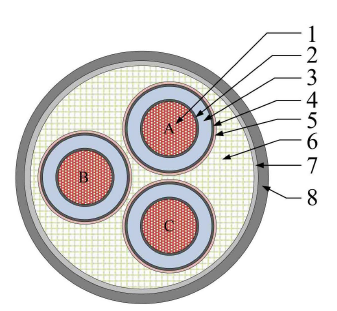Figure 1. Structure diagram of 10 kV three-core HVAC cable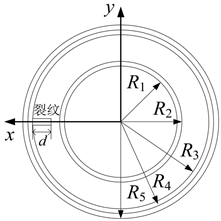Figure 2. Schematic diagram of Single-phase cable size

3.2. 网格剖分和边界条件

1) 网格剖分Table 1. Material parameters of Cable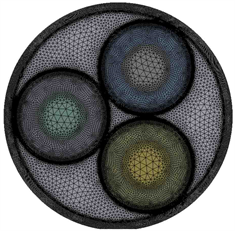Figure 3. Mesh generation

2) 电场边界条件

ABC三相分别施加50 Hz的交流电压和电流激励，Ui = 10 kV，Ii = 325 A，θa = 0，θb = −2π/3，θc = 2π/3。为了防止金属屏蔽层过高的悬浮电压，每一相需要接地，外护套与大地接触，电位也为零，其余均为自然边界条件。

3) 温度边界条件

4. 电-热耦合仿真

4.1. 电–热耦合

$\gamma ={\gamma }_{0}\left(1-{C}_{1}\Delta t-{C}_{2}\Delta {t}^{2}-\cdots -{C}_{n}\Delta {t}^{n}\right)$ (7)

4.2. 模型验证(8)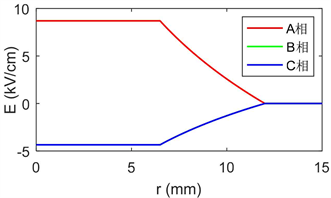Figure 4. Potential radial distribution of three-phase cable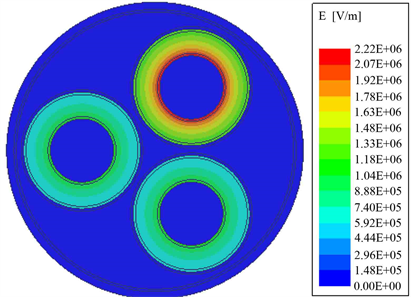Figure 5. Electric field distribution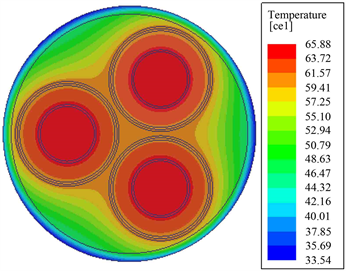Figure 6. Temperature distribution

4.3. 裂纹缺陷分析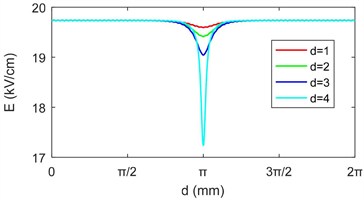Figure 7. Electric field distribution curve of inner surface of insulation under different crack lengths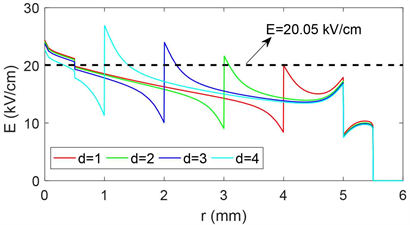Figure 8. Radial electric field distribution curve under different crack lengths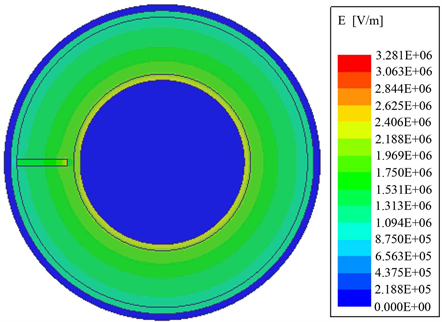Figure 9. Electric field distribution cloud map under air gap defectFigure 10. Temperature distribution under air gap defect

5. 结论

1) d越长，曲线l1上2p处的电场强度减小越快，裂纹气隙中的电场强度迅速上升，d = 4 mm时，气隙电场达到32.8 kV/cm。

2) 当裂缝长度d = 1 mm时，裂纹气隙引起的最大电场等于绝缘内边缘的电场。可以这样认为，当裂纹长度小于1 mm时，电场的增大不易引起局部放电，当裂纹长度大于1 mm时，电场的增大更容易引起局部放电。因此在电缆绝缘制造的时候，考虑到操作电压和雷击过电压等，需要将绝缘内部微小气隙控制到足够小的尺寸。

3) 裂缝气隙引起的持续局部放电会导致电缆绝缘的欧姆损耗增加，导致绝缘温度比正常运行时升高了21.92℃，将会导致电缆额定载流量下降。

  陈茂荣, 杨忠, 牛海清. 中压电缆缺陷原因及其状态检测技术现状[J]. 电线电缆, 2013(5): 39-42.  梁永春. 高压电力电缆温度场和载流量评估研究动态[J]. 高电压技术, 2016, 42(4): 1142-1150.  Rachek, M.H. and Larbi, S.N. (2008) Magnetic Eddy-Current and Thermal Coupled Models for the Finite-Element Behavior Analysis of Underground Power Cables. IEEE Transactions on Magnetics, 44, 4739-4746. https://doi.org/10.1109/TMAG.2008.2004212  Yang, F., Cheng, P., Luo, H., Yang, Y., Liu, H. and Kang, K. (2016) 3-D Thermal Analysis and Contact Resistance Evaluation of Power Cable Joint. Applied Thermal Engineering, 93, 1183-1192. https://doi.org/10.1016/j.applthermaleng.2015.10.076  郝艳捧, 陈云, 阳林, 等. 高压直流海底电缆电–热–流多物理场耦合仿真[J]. 高电压技术, 2017(11): 68-76.  Choo, W., Chen, G. and Swingler, S.G. (2011) Electric Field in Polymeric Cable due to Space Charge Accumulation under DC and Temperature Gradient. IEEE Transactions on Dielectrics and Electrical Insulation, 18, 596-606. https://doi.org/10.1109/TDEI.2011.5739466  李忠华, 刘乐乐, 郑欢, 梁斯婷. HVDC电缆电场分布影响因素的仿真研究[J]. 中国电机工程学报, 2016(9): 2563-2571.  Hjerrild, J., Boggs, S., Holboll, J.T. and Henrik-sen, M. (2001) DC-Field in Solid Dielectric Cables under Transient Thermal Conditions. ICSD’01: Proceedings of the 2001 IEEE 7th International Conference on Solid Dielectrics, Eindhoven, 25-29 June 2001, 58-61.  Lachini, S., Gholami, A. and Mirzaie, M. (2010) The Simulation of Electric Field Distribution on Cable under the Presence of Moisture and Air Voids. Power Engineering & Optimization Conference, Shah Alam, 23-24 June 2010.  Zhu, Z., Chao, H., Ying, Y., Ming, Z. and Qin, Y. (2014) Research on Partial Discharge Mechanism and Characteristics for 10 kV Cable Joint with Air Gap Defect. China International Conference on Electricity Distribution, Shenzhen, 23-26 September 2014. https://doi.org/10.1109/CICED.2014.6991906  陈茂荣, 杨忠, 牛海清. 中压电缆缺陷原因及其状态检测技术现状[J]. 电线电缆, 2013(5): 39-42.  方春华, 汤世祥, 潘明龙. 10 kV电缆中间接头典型缺陷仿真分析[J]. 三峡大学学报(自然科学版), 2016, 38(2): 55-59.